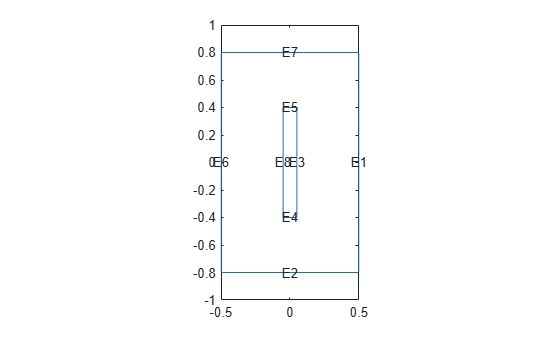# linearizeInput

Specify inputs to linearized model

Since R2021b

## Syntax

``linearizeInput(model,labeltext)``
``input = linearizeInput(model,labeltext)``

## Description

example

````linearizeInput(model,labeltext)` adds inputs for the boundary condition, constraint, load, or source with the label `labeltext`. In the linearized model, the input value u = 1 corresponds to a unit boundary condition acting on the entire region specified by `labeltext`. In other words, simulating the linearized model with the input value u(t) = 25 is equivalent to setting the boundary condition value to 25 in the thermal or structural model in Partial Differential Equation Toolbox™. For more information, see Algorithms.For a structural analysis model, the following boundary conditions, constraints, and loads can become inputs of the linearized model:A structural boundary constraint. Use the `structuralBC` function with the `Constraint` argument.A displacement or a displacement component on the boundary. Use the `structuralBC` function with the `Displacement`, `XDisplacement`, `YDisplacement`, or `ZDisplacement` argument.A structural boundary load. Use the `structuralBoundaryLoad` function with the `Pressure`, `Force`, or `SurfaceTraction` argument.A structural body load. Use the `structuralBodyLoad` function with the `GravitationalAcceleration` argument.The boundary conditions, loads, or constraints with x-, y-, and z- components produce one input channel per component.For a thermal analysis model, the following boundary conditions and sources can become inputs of the linearized model:A temperature or heat flux on the boundary. Use the `thermalBC` function with the `Temperature` or `HeatFlux` argument.An internal heat source. Use the `internalHeatSource` function.Each selected condition or source produces a single scalar input in the linearized model.To make a condition, constraint, load, or source available as a linearization input, always label it upon creation. For example, specify an internal heat source for a thermal model as follows:internalHeatSource(thermalmodel,25,"Label","HeatSource");The remaining boundary conditions are set to zero for linearization purposes, regardless of their value in the structural or thermal model. Ensure that you label all nonzero boundary conditions and pass them as inputs using `linearizeInput`.Use `linearizeInput` and `linearizeOutput` together with the `linearize` function to extract sparse linear models from structural and thermal models.```
````input = linearizeInput(model,labeltext)` returns a structure array `input` with the linearization input description.```

## Examples

collapse all

Use labels to pass the parameters of a 2-D thermal analysis model to the `linearize` function. This function extracts sparse linear models for use with Control System Toolbox™.

Create a transient thermal model.

`thermalmodel = createpde("thermal","transient");`

Add the block geometry to the thermal model by using the `geometryFromEdges` function. The geometry description file for this problem is called `crackg.m`.

`geometryFromEdges(thermalmodel,@crackg);`

Plot the geometry, displaying edge labels.

```pdegplot(thermalmodel,"EdgeLabels","on") ylim([-1,1]) axis equal```Generate a mesh.

`generateMesh(thermalmodel);`

Specify the thermal conductivity, mass density, and specific heat of the material.

```thermalProperties(thermalmodel,"ThermalConductivity",1, ... "MassDensity",1, ... "SpecificHeat",1); ```

Specify the temperature on the left edge as `100`, and constant heat flow to the exterior through the right edge as `-10`. Add a unique label to each boundary condition.

```thermalBC(thermalmodel,"Edge",6,"Temperature",100,"Label","TempBC"); thermalBC(thermalmodel,"Edge",1,"HeatFlux",-10,"Label","FluxBC");```

Specify that the entire geometry generates heat and add a unique label to this assignment.

`internalHeatSource(thermalmodel,25,"Label","HeatSource");`

Set an initial value of `0` for the temperature.

`thermalIC(thermalmodel,0);`

Call the `linearizeInput` function with the previously defined labels for the boundary conditions and the internal heat source to set the inputs for the `linearize` function. Add one label per function call.

```linearizeInput(thermalmodel,"HeatSource"); linearizeInput(thermalmodel,"TempBC"); linearizeInput(thermalmodel,"FluxBC");```

The `LinearizeInputs` property of `thermalmodel` stores the inputs.

`thermalmodel.LinearizeInputs`
```ans=1×3 struct array with fields: RegionType RegionID Label ```

Create inputs for gravity and a short pressure pulse on tuning fork.

Create a structural transient analysis model.

`structuralmodel = createpde("structural","transient-solid");`

Import and plot the tuning fork geometry.

```importGeometry(structuralmodel,"TuningFork.stl"); pdegplot(structuralmodel)```Generate a mesh.

`generateMesh(structuralmodel,"Hmax",0.005);`

Specify Young's modulus, Poisson's ratio, and the mass density to model linear elastic material behavior. Specify all physical properties in consistent units.

```structuralProperties(structuralmodel,"YoungsModulus",210E9, ... "PoissonsRatio",0.3, ... "MassDensity",8000);```

Identify faces for applying boundary constraints and loads by plotting the geometry with the face labels.

```figure("units","normalized","outerposition",[0 0 1 1]) pdegplot(structuralmodel,"FaceLabels","on") view(-50,15) title("Geometry with Face Labels")```Impose sufficient boundary constraints to prevent rigid body motion under applied loading. Typically, you hold a tuning fork by hand or mount it on a table. As a simplified approximation of this boundary condition, fix a region near the intersection of the tines and the handle (faces 21 and 22).

`structuralBC(structuralmodel,"Face",[21,22],"Constraint","fixed");`

Specify the pressure loading on a tine as a short rectangular pressure pulse.

```structuralBoundaryLoad(structuralmodel,"Face",11, ... "Pressure",5E6, ... "EndTime",1e-3, ... "Label","Pressure");```

Specify the acceleration due to gravity as a body load.

```structuralBodyLoad(structuralmodel, ... "GravitationalAcceleration",[0 0 -1], ... "Label","Gravity");```

Create inputs for gravity and the pressure pulse on the tuning fork.

```linearizeInput(structuralmodel,"Gravity"); linearizeInput(structuralmodel,"Pressure");```

The `LinearizeInputs` property of `structuralmodel` stores the inputs.

`structuralmodel.LinearizeInputs`
```ans=1×2 struct array with fields: RegionType RegionID Label ```

## Input Arguments

collapse all

Structural or linear thermal model, specified as a `StructuralModel` object or a `ThermalModel` object. The `linearize` function does not support nonlinear thermal analysis.

Label for boundary condition, specified as a character vector or a string.

Data Types: `char` | `string`

## Output Arguments

collapse all

Linearization input description, returned as a structure array.

## Algorithms

The `linearize` function constructs a linear model whose inputs are a subset of the boundary conditions, loads, or sources applied to the thermal or structural model in Partial Differential Equation Toolbox and whose outputs are the resulting values at the selected DoFs. For example, if you designate the heat source

`internalHeatSource(model,25,"Face",2,"Label","heatSource")`

as a linearization input

`linearizeInput(model,"heatSource")`

and designate the temperatures on face `X` as linearization outputs

`linearizeOutput(model,"Face",X)`
then the response of the linearized model to the constant input u(t) = 25 (the heat source value in the thermal model) matches the Partial Differential Equation Toolbox simulation results for face `X`.

```tlist = 1:10; u = repmat(25,size(tlist)); ysp = lsim(linsys,uLoad,tlist);```

Note that loads and boundary conditions not included as linearization inputs are assumed to be zero in the linearized model regardless of their values in the structural or thermal model in Partial Differential Equation Toolbox. Simulation results can differ in this case.

## Version History

Introduced in R2021b